# Fitting a PHA Data Set with Multiple Responses## Overview

#### Synopsis:

This thread demonstrates the use of the Sherpa functions set_full_model, set_bkg_full_model, and get_response to explicitly define complex source and background model expressions in which some of the model components are convolved with the instrument response, while others are not. It is not possible to define such a model expression in the usual way with set_source and set_bkg_source, as these functions do not allow for applying separate responses to individual model components within a single model expression.

We consider the scenario in which one would choose to model the X-ray background together with the source emission, instead of subtracting it. For example, this would be useful for modeling extended, diffuse source emission which covers the entire field of view of an observation, thereby leaving no source-free region from which to extract a background spectrum. As a result, the various components contributing to the background level would be modeled together with the source emission (assuming that using an average background level from a blank-sky data set is inappropriate).

If you would like to fit a background-subtracted source spectrum using a common RMF and ARF for the source and background, or fit source and background spectra simultaneously with proper and distinct RMFs and ARFs (but with one response per model expression, unlike in this thread), refer to the Sherpa thread Simultaneously Fitting Source and Background Spectra.

The sample data files used in this thread are available in sherpa.tar.gz; they can be generated by following the CIAO thread Using specextract to Extract ACIS Spectra and Response Files for Extended Sources .

Last Update: 8 Dec 2022 - Updated for CIAO 4.15, removed references to ChIPS.

## Reading the PHA Data into Sherpa

In this thread, we wish to fit the the PHA spectrum contained in the FITS file source.pi. This data set is read into Sherpa with the load_pha command, and assigned to default data set ID "1":

```sherpa> load_pha("source.pi")
```

The associated background PHA file and ARF and RMF instrument response files are automatically read into the session along with the source data by the load_pha function. If the names of these files had not been recorded in the header of source.pi, they could have been loaded with load_bkg, load_arf, and load_rmf.

Information on all loaded data sets may be viewed with the show_all command, or individually with show_data, show_bkg, print(get_arf())/print(get_rmf()), and print(get_bkg_arf())/print(get_bkg_rmf()). The source, background, and source ARF data may be visualized with plot_data, plot_bkg and plot_arf (or simply the plot command, as shown below).

```sherpa> set_xlog()
sherpa> set_ylog()

sherpa> ignore(None, 1)
dataset 1: 0.0073:14.9504 -> 1.0074:14.9504 Energy (keV)

sherpa> ignore(7, None)
dataset 1: 1.0074:14.9504 -> 1.0074:6.9934 Energy (keV)

sherpa> group_counts(1, 30, bkg_id=1)
sherpa> group_counts(1, 30)

sherpa> plot("data", "bkg")
```

Before plotting, we set our preferences for the x- and y-axis scale of all data, model, and fit plots created in this session to logarithmic by using the set_xlog and set_ylog commands with no arguments (to learn how to change the default axis scale from linear to logarithmic so that these commands do not have to be run in each Sherpa session, see this Sherpa FAQ). Then, we filter the data with the ignore command in order to include only the 1.0-7.0 keV range in our analysis, and group both spectra so that each bin contains a minumum of 30 counts to ensure that the spectral features are clearly visible in the plot displayed in Figure 1. Finally, the source and background data sets are plotted in separate plots within one window using the plot command.

### Figure 1: Plot of PHA source and background spectra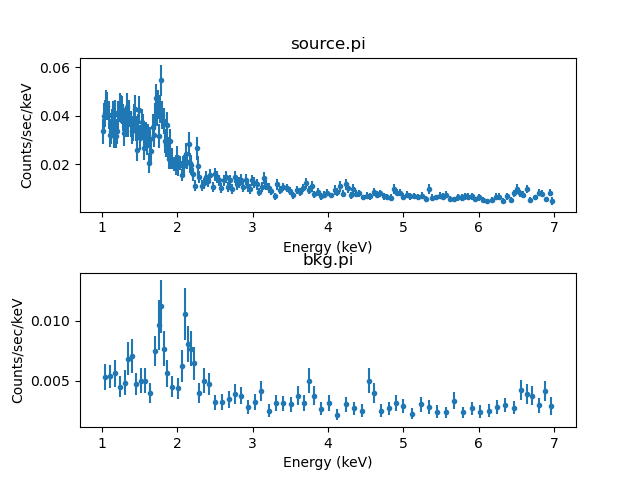### Figure 1: Plot of PHA source and background spectra

The grouped source spectrum (top) and associated background spectrum (bottom).

After plotting the spectra we ungroup the data sets, since we wish to fit the data ungrouped.

```sherpa> ungroup()
```

## Defining the Instrument Responses

### Source Instrument Response

The ARF and RMF response files for source.pi were automatically loaded with load_pha; therefore, we do not have to load them separately with load_arf and load_rmf. We assign the instrument response associated with the source data to the variable 'rsp' using the get_response function, which returns the instrument response model (ARF*RMF) associated with a data set.

```sherpa> rsp = get_response()
```

The source data set ID is "1", which is the default, therefore it is not necessary to explicitly enter a data set ID as an argument to get_response. The current definition of the instrument responses may be examined using show_all or the get_arf/get_bkg_arf and get_rmf/get_bkg_rmf commands:

```sherpa> print(get_arf())
name     = source.warf
energ_lo = Float64
energ_hi = Float64
specresp = Float64
bin_lo   = None
bin_hi   = None
exposure = 110163.23967791
ethresh  = 1e-10

sherpa> print(get_rmf())
name     = source.wrmf
energ_lo = Float64
energ_hi = Float64
n_grp    = UInt64
f_chan   = UInt32
n_chan   = UInt32
matrix   = Float64
e_min    = Float64
e_max    = Float64
detchans = 1024
offset   = 1
ethresh  = 1e-10

sherpa> print(get_bkg_arf())
name     = bkg.warf
energ_lo = Float64
energ_hi = Float64
specresp = Float64
bin_lo   = None
bin_hi   = None
exposure = 110163.23967791
ethresh  = 1e-10

sherpa> print(get_bkg_rmf())
name     = bkg.wrmf
energ_lo = Float64
energ_hi = Float64
n_grp    = UInt64
f_chan   = UInt32
n_chan   = UInt32
matrix   = Float64
e_min    = Float64
e_max    = Float64
detchans = 1024
offset   = 1
ethresh  = 1e-10
```

The output shows that source.warf, source.wrmf, bkg.warf, and bkg.wrmf currently define the source and background instrument responses.

### Background Instrument Responses

In this example, we choose to model the background emission together with the source emission, which in this case involves defining a complex background model consisting of multiple components. We assume that the same ARF and RMF associated with the source data set apply to the background spectrum (i.e., there is not an independent response for the background since the background spectrum was extracted from a source-free region of the source observation).

The background level of an X-ray observation consists of contributions from both astrophysical and instrumental sources; therefore, some components of the background model need to be convolved with the source instrument response, whereas others do not. This requires that the instrumental components of the background model be unconvolved, i.e. convolved with a unit effective area, defined as follows:

```sherpa> copy_data(1, 2)

sherpa> unit_arf = get_bkg_arf(2)
sherpa> unit_arf.specresp = np.ones_like(unit_arf.specresp)

sherpa> bunitrsp = get_response(2, bkg_id=1)
```

Here, we simply copy source data set 1 and its associated background and responses (id=1, bkg_id=1) to data set 2 (id=2, bkg_id=1) to avoid overwriting the background ARF data contained in data set 1, which we will need for the background model components which should be folded through the instrument effective area. Then, we assign the background ARF model in data set 2 (which is exactly the same as the background ARF in data set 1) returned by get_arf to the variable 'unit_arf' and set the spectral response of 'unit_arf' to contain all 1s. This is the unit ARF response model which is appropriate for convolving the instrumental background components of the model expression. The ARF*RMF instrument response model stored in variable 'bunitrsp' contains the unit background ARF and the untouched background RMF.

The background ARF*RMF instrument response model returned by 'get_response(bkg_id=1)' should be used to convolve the cosmic background model component, as shown in the next section. We define another response variable for the background, 'brsp', which includes the untouched ARF and untouched RMF for convolving the astrophysical background component:

```sherpa> brsp = get_response(bkg_id=1)
```

## Defining the Model Expressions

### Background Model

The set_full_model and set_bkg_full_model functions are used to explicitly define complex source and background model expressions for simultaneous fitting. Since the background model in this example is complex, in that it contains many model components, each convolved by a separate response, we consider the background model components first.

We assume that the background contributions to the emission spectrum consist of an absorbed thermal plasma model to represent the astrophysical diffuse Cosmic X-ray Background (CXB), and several Gaussian models plus an exponential cutoff power-law model to fit the quiescent instrumental background.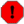Warning

This example does not fully account for all possible background contributions to a Chandra ACIS data set—e.g., we are ignoring the contribution from resolved point sources to the CXB—it is meant only for demonstration purposes.

We define the explicit background model expression using the set_bkg_full_model function, which is used to model the background associated with a source modeled by the set_full_model function. Note that it is not possible to define such a complex background model expression in the usual way with set_bkg_source, as this function does not allow for applying separate responses to individual model components within a single model expression.

```sherpa> mdl1 = gauss1d.bg1 + gauss1d.bg2 + xscutoffpl.bpl
sherpa> mdl2 = xsphabs.gal * xsapec.bth
sherpa> set_bkg_full_model(bunitrsp(mdl1) + brsp(mdl2))
```

Multiplication by the background exposure time is implicit, and the necessary scaling resulting from differences between the source and background exposure times and spectral extraction areas is applied to the background model within the set_full_model expression, as shown in the next section (note in this example, the exposure ratio is 1 since the source and background spectra were extracted from the same observation).

### Source Model

To describe the source contributions to the total emission in the spectrum, we define a source model consisting of an absorbed power-law multiplied by an absorbed thermal plasma model, to describe the bright central point source and the surrounding diffuse thermal gas, respectively. We specify that the source model be convolved by the instrument response contained in the variable 'rsp', defined above. Multiplication by the source exposure time is done implicitly. We define the full model expression to include the source and background components, including the scaling of the background to account for the different spectral extraction areas of the source and background.

```sherpa> my_bkg_model = (bunitrsp(mdl1) + brsp(mdl2))
sherpa> scale = get_bkg_scale()
sherpa> print(scale)
1.8366082517267892

sherpa> smdl = (xsphabs.gal + xsphabs.intrin) * xspowerlaw.spl + gal*xsapec.sth
sherpa> set_full_model(rsp(smdl) + scale * my_bkg_model)
```

Note that the source component in the set_full_model expression above would normally be defined by doing 'set_source((xsphabs.gal+xsphabs.intrin)*xspowerlaw.spl + gal*xsapec.sth)', which automatically and implicitly convolves the source model expression with the appropriate response. We do not define the source model in this usual way in this example, since the associated background spectrum requires a complex model expression consisting of multiple components convolved by different responses. Otherwise, set_bkg_source would be used to set the background source model in the usual way, for simultaneous source and background fitting.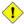Caution

It is important to keep in mind that the Sherpa functions which are related to a source or background model defined with set_source or set_bkg_source, such as plot_source/plot_bkg_source or calc_energy_flux, are not compatible with the complete model expression defined by the set_full_model or set_bkg_full_model functions. In order to use these Sherpa functions, source and background models should be defined in the usual way with the automatic functions set_source and set_bkg_source.

## Modifying Method & Statistic Settings

The show_method and show_stat functions may be used to view the current method and statistics settings:

```sherpa> show_method()
Optimization Method: LevMar
name     = levmar
ftol     = 1.1920928955078125e-07
xtol     = 1.1920928955078125e-07
gtol     = 1.1920928955078125e-07
maxfev   = None
epsfcn   = 1.1920928955078125e-07
factor   = 100.0
verbose  = 0

sherpa> show_stat()
Statistic: Chi2Gehrels
Chi Squared with Gehrels variance.

The variance is estimated from the number of counts in each bin,
but unlike `Chi2DataVar`, the Gaussian approximation is not
used. This makes it more-suitable for use with low-count data.

The standard deviation for each bin is calculated using the
approximation from _:

sigma(i,S) = 1 + sqrt(N(i,s) + 0.75)

where the higher-order terms have been dropped. This is accurate
to approximately one percent. For data where the background has
not been subtracted then the error term is:

sigma(i) = sigma(i,S)

whereas with background subtraction,

sigma(i)^2 = sigma(i,S)^2 + [A(S)/A(B)]^2 sigma(i,B)^2

A(B) is the off-source "area", which could be
the size of the region from which the background is extracted, or
the length of a background time segment, or a product of the two,
etc.; and A(S) is the on-source "area". These terms may be defined
for a particular type of data: for example, PHA data sets A(B) to
`BACKSCAL * EXPOSURE` from the background data set and A(S) to
`BACKSCAL * EXPOSURE` from the source data set.

--------
Chi2DataVar, Chi2ModVar, Chi2XspecVar

Notes
-----
The accuracy of the error term when the background has been
subtracted has not been determined. A preferable approach to
background subtraction is to model the background as well as the
source signal.

References
----------

..  "Confidence limits for small numbers of events in
astrophysical data", Gehrels, N. 1986, ApJ, vol 303,
p. 336-346.
```

We decide to use the Cstat fit statistic and Nelder-Mead optimization method for fitting this data, which we specify using the set_stat and set_method commands.

```sherpa> set_method("neldermead")
sherpa> set_stat("cstat")
```

Details about the optimization methods and fit statistics available in Sherpa may be accessed by typing 'ahelp [method name]' at the Sherpa prompt, or by visiting the Sherpa Statistics and Optimization Methods pages.

```sherpa> ahelp "levmar"
sherpa> ahelp "chi2datavar"
```

Note that it is usually advisable to switch the optimization method and/or fit statistic at least once during a fitting trial to get a feel for the model parameter space and try to determine the "true" best-fit model parameters. Observe an example of this in the Fitting section below, where we first fit the background model using the chosen Nelder-Mead optimization method. To fit the full source and background model, the Levenberg-Marquardt method is used before returning to Nelder-Mead to see how this affects the fit results. The Sherpa Optimization Methods page contains a detailed comparison of the available methods in Sherpa.

## Fitting

The current definition of the Sherpa source model expression, including initial parameter values for each model component, may be examined using show_model:

```sherpa> show_model()
Model: 1
(apply_rmf(apply_arf((110163.23967791 * (((xsphabs.gal + xsphabs.intrin) * xspowerlaw.spl) + (xsphabs.gal * xsapec.sth))))) + (1.8366082517267892 * (apply_rmf(apply_arf((110163.23967791 * ((gauss1d.bg1 + gauss1d.bg2) + xscutoffpl.bpl)))) + apply_rmf(apply_arf((110163.23967791 * (xsphabs.gal * xsapec.bth)))))))
Param        Type          Value          Min          Max      Units
-----        ----          -----          ---          ---      -----
gal.nH       thawed            1            0        1e+06 10^22 atoms / cm^2
intrin.nH    thawed            1            0        1e+06 10^22 atoms / cm^2
spl.PhoIndex thawed            1           -3           10
spl.norm     thawed            1            0        1e+24
sth.kT       thawed            1        0.008           64        keV
sth.Abundanc frozen            1            0            5
sth.redshift frozen            0       -0.999           10
sth.norm     thawed            1            0        1e+24
bg1.fwhm     thawed           10  1.17549e-38  3.40282e+38
bg1.pos      thawed            0 -3.40282e+38  3.40282e+38
bg1.ampl     thawed            1 -3.40282e+38  3.40282e+38
bg2.fwhm     thawed           10  1.17549e-38  3.40282e+38
bg2.pos      thawed            0 -3.40282e+38  3.40282e+38
bg2.ampl     thawed            1 -3.40282e+38  3.40282e+38
bpl.PhoIndex thawed            1           -3           10
bpl.HighECut thawed           15         0.01          500        keV
bpl.norm     thawed            1            0        1e+24
bth.kT       thawed            1        0.008           64        keV
bth.Abundanc frozen            1            0            5
bth.redshift frozen            0       -0.999           10
bth.norm     thawed            1            0        1e+24
```

Since we are fitting a complex model expression to the data containing multiple components and responses, we approach the final fit to the data in a series of smaller trials in which we fit each model component separately, before fitting the entire model.

### Background

The Sherpa guess function may be used to estimate initial parameter values for a model, as well as the minima and maxima for their ranges. To have Sherpa automatically query for the initial parameter values when a model is established, set 'paramprompt(True)' (it is 'False' by default).

We guess the initial parameter values for some background model components and set other values ourselves. For the thermal plasma model, we assume the Solar abundances contained in Grevesse, N. & Sauval, A.J. (1998, Space Science Reviews 85, 161).

```sherpa> set_xsabund("grsa")
Solar Abundance Vector set to grsa:  Grevesse, N. & Sauval, A. J. Space Science Reviews 85, 161 (1998)
```
```sherpa> guess(bpl)
sherpa> guess(bg1)
sherpa> guess(bg2)
sherpa> guess(bth)

sherpa> set_par(gal.nH, 0.041, frozen=True)

sherpa> bpl.phoindex = 0.15
sherpa> bpl.HighECut = 5.6

sherpa> bg1.pos = 1.77
sherpa> bg2.pos= 2.15

sherpa> bth.kT = 0.15
```

First, we fit only the instrumental power-law component using the fit_bkg function, which fits only the background data set(s) current in the session; this requires temporarily re-defining the background model expression to include only the model component we are interested in fitting:

```sherpa> set_bkg_full_model(bunitrsp(bpl))
sherpa> fit_bkg(1)
Dataset               = 1
Statistic             = cstat
Initial fit statistic = 824.024
Final fit statistic   = 583.812 at function evaluation 555
Data points           = 412
Degrees of freedom    = 409
Probability [Q-value] = 2.78352e-08
Reduced statistic     = 1.42741
Change in statistic   = 240.212
bpl.PhoIndex   0.464138
bpl.HighECut   499.986
bpl.norm       0.00648035
```

Next, we successively add the two instrumental Gaussian model components to the background model expression, and finally the cosmic thermal plasma component, re-fitting at each step:

```sherpa> set_bkg_full_model(bunitrsp(bpl + bg1))
sherpa> fit_bkg(1)
Dataset               = 1
Statistic             = cstat
Initial fit statistic = 564.839
Final fit statistic   = 533.127 at function evaluation 1690
Data points           = 412
Degrees of freedom    = 406
Probability [Q-value] = 2.17032e-05
Reduced statistic     = 1.31312
Change in statistic   = 31.7119
bpl.PhoIndex   0.417642
bpl.HighECut   498.928
bpl.norm       0.00592858
bg1.fwhm       0.0444975
bg1.pos        2.14755
bg1.ampl       0.0164747

sherpa> set_bkg_full_model(bunitrsp(mdl1))
sherpa> fit_bkg(1)
Dataset               = 1
Statistic             = cstat
Initial fit statistic = 563.307
Final fit statistic   = 491.238 at function evaluation 3160
Data points           = 412
Degrees of freedom    = 403
Probability [Q-value] = 0.00169764
Reduced statistic     = 1.21895
Change in statistic   = 72.069
bg1.fwhm       0.0252809
bg1.pos        1.75945
bg1.ampl       0.0246506
bg2.fwhm       0.0243767
bg2.pos        2.14768
bg2.ampl       0.0316482
bpl.PhoIndex   0.349091
bpl.HighECut   368.203
bpl.norm       0.00530339

sherpa> set_bkg_full_model(bunitrsp(mdl1) + brsp(mdl2))
sherpa> fit_bkg(1)

Dataset               = 1
Statistic             = cstat
Initial fit statistic = 1892.13
Final fit statistic   = 489.71 at function evaluation 2886
Data points           = 412
Degrees of freedom    = 401
Probability [Q-value] = 0.00158638
Reduced statistic     = 1.22122
Change in statistic   = 1402.42
bg1.fwhm       0.0148916
bg1.pos        1.759
bg1.ampl       0.0434772
bg2.fwhm       0.0648597
bg2.pos        2.14838
bg2.ampl       0.0127508
bpl.PhoIndex   0.309787
bpl.HighECut   373.928
bpl.norm       0.00499216
bth.kT         0.277593
bth.norm       6.53235e-06
```

We can check that the fit was successful by examining the fit residuals (Figure 2):

```sherpa> plot_bkg_fit_resid(yerrorbars=False)
sherpa> plt.yscale('linear')
```

### Figure 2: Background fit with residuals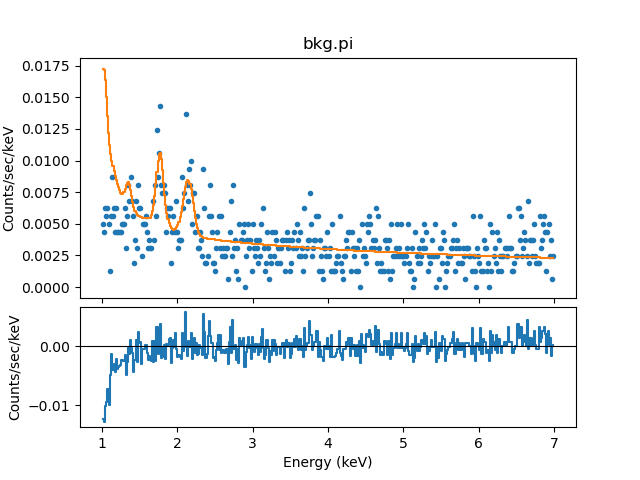### Source plus background

Now that we have reasonable background model parameter values resulting from the background fitting trials, we can fit the entire source-plus-background model expression, first with the background model parameters frozen to constrain the source model, and finally with them thawed.

We fit the source and background model components together in one fitting trial after setting initial model parameter values for the source:

```sherpa> show_bkg_model(1)
Background Model: 1:1
(apply_rmf(apply_arf((110163.23967791 * ((gauss1d.bg1 + gauss1d.bg2) + xscutoffpl.bpl)))) + apply_rmf(apply_arf((110163.23967791 * (xsphabs.gal * xsapec.bth)))))
Param        Type          Value          Min          Max      Units
-----        ----          -----          ---          ---      -----
bg1.fwhm     thawed    0.0148916    0.0001314        131.4
bg1.pos      thawed        1.759       1.0074       7.0226
bg1.ampl     thawed    0.0434772  6.37784e-06      6.37784
bg2.fwhm     thawed    0.0648597    0.0001314        131.4
bg2.pos      thawed      2.14838       1.0074       7.0226
bg2.ampl     thawed    0.0127508  6.37784e-06      6.37784
bpl.PhoIndex thawed     0.309787           -3           10
bpl.HighECut thawed      373.928         0.01          500        keV
bpl.norm     thawed   0.00499216  6.37784e-06      6.37784
gal.nH       frozen        0.041            0        1e+06 10^22 atoms / cm^2
bth.kT       thawed     0.277593        0.008           64        keV
bth.Abundanc frozen            1            0            5
bth.redshift frozen            0       -0.999           10
bth.norm     thawed  6.53235e-06  6.37784e-06      6.37784

sherpa> show_model(1)
Model: 1
(apply_rmf(apply_arf((110163.23967791 * (((xsphabs.gal + xsphabs.intrin) * xspowerlaw.spl) + (xsphabs.gal * xsapec.sth))))) + (1.8366082517267892 * (apply_rmf(apply_arf((110163.23967791 * ((gauss1d.bg1 + gauss1d.bg2) + xscutoffpl.bpl)))) + apply_rmf(apply_arf((110163.23967791 * (xsphabs.gal * xsapec.bth)))))))
Param        Type          Value          Min          Max      Units
-----        ----          -----          ---          ---      -----
gal.nH       frozen        0.041            0        1e+06 10^22 atoms / cm^2
intrin.nH    thawed            1            0        1e+06 10^22 atoms / cm^2
spl.PhoIndex thawed            1           -3           10
spl.norm     thawed            1            0        1e+24
sth.kT       thawed            1        0.008           64        keV
sth.Abundanc frozen            1            0            5
sth.redshift frozen            0       -0.999           10
sth.norm     thawed            1            0        1e+24
bg1.fwhm     thawed    0.0148916    0.0001314        131.4
bg1.pos      thawed        1.759       1.0074       7.0226
bg1.ampl     thawed    0.0434772  6.37784e-06      6.37784
bg2.fwhm     thawed    0.0648597    0.0001314        131.4
bg2.pos      thawed      2.14838       1.0074       7.0226
bg2.ampl     thawed    0.0127508  6.37784e-06      6.37784
bpl.PhoIndex thawed     0.309787           -3           10
bpl.HighECut thawed      373.928         0.01          500        keV
bpl.norm     thawed   0.00499216  6.37784e-06      6.37784
bth.kT       thawed     0.277593        0.008           64        keV
bth.Abundanc frozen            1            0            5
bth.redshift frozen            0       -0.999           10
bth.norm     thawed  6.53235e-06  6.37784e-06      6.37784

sherpa> freeze(bpl, bg1, bg2, bth.norm, bth.kT)
sherpa> guess(spl)
sherpa> guess(sth)
sherpa> intrin.nH = 0.038
sherpa> spl.phoindex = 2.0
sherpa> set_par(sth.redshift, 2.0, frozen=True)
sherpa> sth.kT = 1.5
```

The source and background data assigned to data set 1 may be simultaneously fit using the fit function, as follows:

```sherpa> set_method("levmar")
sherpa> fit(1)
Dataset               = 1
Method                = levmar
Statistic             = cstat
Initial fit statistic = 1.14397e+06
Final fit statistic   = 921.131 at function evaluation 273
Data points           = 824
Degrees of freedom    = 819
Probability [Q-value] = 0.0073046
Reduced statistic     = 1.1247
Change in statistic   = 1.14305e+06
intrin.nH      1.09259      +/- 1.00164
spl.PhoIndex   2.06018      +/- 0.97261
spl.norm       6.40215e-06  +/- 1.32253e-05
sth.kT         38.0081      +/- 0
sth.norm       0.00130525   +/- 0.000887582

sherpa> thaw(bpl, bg1, bg2, bth.norm, bth.kT)
sherpa> fit(1)
Dataset               = 1
Method                = levmar
Statistic             = cstat
Initial fit statistic = 921.131
Final fit statistic   = 905.132 at function evaluation 726
Data points           = 824
Degrees of freedom    = 808
Probability [Q-value] = 0.0095871
Reduced statistic     = 1.12021
Change in statistic   = 15.9988
intrin.nH      0.75837      +/- 7.28346
spl.PhoIndex   1.9358       +/- 2.30116
spl.norm       7.35352e-06  +/- 3.49273e-05
sth.kT         27.566       +/- 0
sth.norm       0.00120724   +/- 0.000800217
bg1.fwhm       0.0177675    +/- 0.0841763
bg1.pos        1.75778      +/- 0.00587382
bg1.ampl       0.0495421    +/- 0.231662
bg2.fwhm       0.0510932    +/- 0.0503858
bg2.pos        2.14748      +/- 0.00871347
bg2.ampl       0.0133648    +/- 0.0122892
bpl.PhoIndex   0.268548     +/- 0.044806
bpl.HighECut   500          +/- 0
bpl.norm       0.00483035   +/- 0.000332127
bth.kT         0.235494     +/- 0.993207
bth.norm       6.37784e-06  +/- 0.000109177
WARNING: parameter value bpl.HighECut is at its maximum boundary 500.0
WARNING: parameter value bth.norm is at its minimum boundary 6.377838630420506e-06

sherpa> fit(1)
Dataset               = 1
Statistic             = cstat
Initial fit statistic = 905.132
Final fit statistic   = 897.606 at function evaluation 5679
Data points           = 824
Degrees of freedom    = 808
Probability [Q-value] = 0.0150786
Reduced statistic     = 1.1109
Change in statistic   = 7.52627
intrin.nH      0.843044
spl.PhoIndex   1.89341
spl.norm       5.1876e-05
sth.kT         12.4368
sth.norm       6.38001e-06
bg1.fwhm       0.00655948
bg1.pos        1.7585
bg1.ampl       0.129364
bg2.fwhm       0.0255401
bg2.pos        2.14882
bg2.ampl       0.0263915
bpl.PhoIndex   0.25552
bpl.HighECut   406.11
bpl.norm       0.00477846
bth.kT         0.130059
bth.norm       0.00012421
```

The plot_fit_resid and plot_fit_delchi functions may be used to visualize the quality of the fit, and the Matplotlib plt.savefig function to save the plot as a PostScript file (or a PNG one, as shown in ):

```sherpa> plot_fit_resid()
WARNING: The displayed errorbars have been supplied with the data or calculated using chi2xspecvar; the errors are not used in fits with cstat
WARNING: The displayed errorbars have been supplied with the data or calculated using chi2xspecvar; the errors are not used in fits with cstat
sherpa> plt.yscale('linear')
sherpa> plt.savefig("sherpa_fit.ps")
```

### Figure 3: Source-plus-background fit with residuals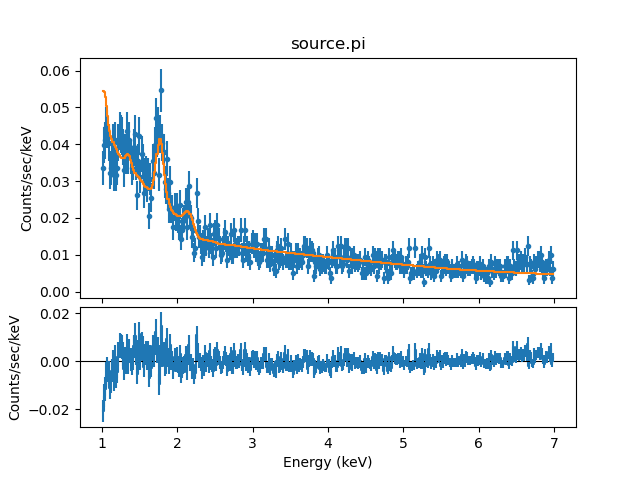## Plotting individual fitted model components

To visualize the contributions to the fit of the emission from the individual source and background components, we can use the Sherpa plot_model_component command.

```sherpa> plot_fit()
sherpa> plot_model_component(rsp(gal * spl), overplot=True)
sherpa> plot_model_component(bunitrsp(bpl), overplot=True)
sherpa> plot_model_component(brsp(gal * bth), overplot=True)
sherpa> plt.ylim(1e-4, 0.1)
sherpa> plt.title("Source and background model components")
```

The resulting plot is shown in Figure 4

### Figure 4: Individual components of best-fit model to source and background spectra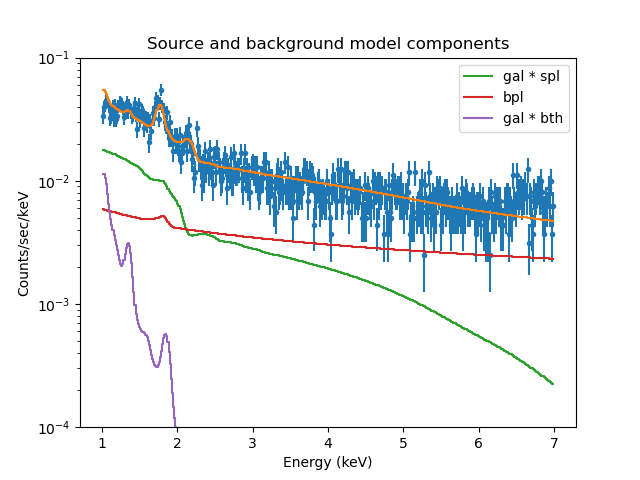### Figure 4: Individual components of best-fit model to source and background spectra

Plot of the best-fit model to the combined source and background spectra in red, with the individual model components overlaid in different colors.

As demonstrated above, the plot_model_component command may be used to plot one, or a combination of, individual source model components to allow the user to quickly visualize the contribution to the full model being used to fit the data. The model components plotted with this command are convolved with any assigned convolution models, e.g. PSF or PHA responses. The plot_source_component command is available for visualizing the unconvolved model components, and get_model_component_plot/get_source_component_plot for accessing the data arrays and preferences which define the corresponding plot.

## Examining Fit Results

Several algorithms are available in Sherpa for examining fit results, such as confidence, covariance, interval-projection, and region-projection. Here, we use the preferred confidence method to estimate the 1σ confidence intervals for the thawed model parameters.

```sherpa> set_conf_opt("fast","True")

sherpa> conf(1)
...
{verbose output omitted for brevity}
...

Dataset               = 1
Confidence Method     = confidence
Iterative Fit Method  = None
Statistic             = cstat
confidence 1-sigma (68.2689%) bounds:
Param            Best-Fit  Lower Bound  Upper Bound
-----            --------  -----------  -----------
intrin.nH        0.843044    -0.262741     0.444752
spl.PhoIndex      1.89341    -0.205211     0.106904
spl.norm       5.1876e-05 -1.43197e-05   8.6116e-06
sth.kT            12.4368        -----        -----
sth.norm      6.38001e-06        -----   0.00029798
bg1.fwhm       0.00655948  -0.00644453    0.0927277
bg1.pos            1.7585   -0.0112509   0.00875073
bg1.ampl         0.129364    -0.120221      1.55857
bg2.fwhm        0.0255401   -0.0254475    0.0934451
bg2.pos           2.14882   -0.0112512    0.0087509
bg2.ampl        0.0263915   -0.0195163     0.277166
bpl.PhoIndex      0.25552   -0.0739628    0.0479101
bpl.HighECut       406.11     -323.265        -----
bpl.norm       0.00477846  -0.00044798  0.000373317
bth.kT           0.130059        -----     0.250027
bth.norm       0.00012421        -----   0.00307748
```

Note that the lines of dashes "----" in the confidence results indicate that the hard upper or lower limit was reached for one or more parameters. This occurs when the parameter boundary found by the confidence method lies outside the hard limit boundary for a model parameter—this could result from an issue with the signal-to-noise of the data, the applicability of the model to the data, systematic errors in the data, among other things. The interval-projection and region-projection routines are useful for exploring these issues further.

The quality of a fit is also available in the χ2 goodness-of-fit information reported after each fit, as well as in the output of the functions get_fit_results and show_fit .

```sherpa> show_fit()
name         = simplex
ftol         = 1.1920928955078125e-07
maxfev       = None
initsimplex  = 0
finalsimplex = 9
step         = None
verbose      = 0
reflect      = True

Statistic: CStat
Maximum likelihood function (XSPEC style).

This is equivalent to the XSpec implementation of the
Cash statistic _ except that it requires a model to be fit
to the background. To handle the background in the same manner
as XSpec, use the WStat statistic.

Counts are sampled from the Poisson distribution, and so the best
way to assess the quality of model fits is to use the product of
individual Poisson probabilities computed in each bin i, or the
likelihood L:

L = (product)_i [ M(i)^D(i)/D(i)! ] * exp[-M(i)]

where M(i) = S(i) + B(i) is the sum of source and background model
amplitudes, and D(i) is the number of observed counts, in bin i.

The cstat statistic is derived by (1) taking the logarithm of the
likelihood function, (2) changing its sign, (3) dropping the
factorial term (which remains constant during fits to the same
dataset), (4) adding an extra data-dependent term (this is what
makes it different to `Cash`, and (5) multiplying by two:

C = 2 * (sum)_i [ M(i) - D(i) + D(i)*[log D(i) - log M(i)] ]

The factor of two exists so that the change in the cstat statistic
from one model fit to the next, (Delta)C, is distributed
approximately as (Delta)chi-square when the number of counts in
each bin is high.  One can then in principle use (Delta)C instead
of (Delta)chi-square in certain model comparison tests. However,
unlike chi-square, the cstat statistic may be used regardless of
the number of counts in each bin.

The inclusion of the data term in the expression means that,
unlike the Cash statistic, one can assign an approximate
goodness-of-fit measure to a given value of the cstat statistic,
i.e. the observed statistic, divided by the number of degrees of
freedom, should be of order 1 for good fits.

Notes
-----
The background should not be subtracted from the data when this
statistic is used.  It should be modeled simultaneously with the
source.

The cstat statistic function evaluates the logarithm of each data
point. If the number of counts is zero or negative, it's not
possible to take the log of that number. The behavior in this case
is controlled by the `truncate` and `trunc_value` settings in the
.sherpa.rc file:

- if `truncate` is `True` (the default value), then
`log(trunc_value)` is used whenever the data value is <= 0.  The
default is `trunc_value=1.0e-25`.

- when `truncate` is `False` an error is raised.

References
----------

..  The description of the Cash statistic (`cstat`) in

Fit:Dataset               = 1
Statistic             = cstat
Initial fit statistic = 905.132
Final fit statistic   = 897.606 at function evaluation 5679
Data points           = 824
Degrees of freedom    = 808
Probability [Q-value] = 0.0150786
Reduced statistic     = 1.1109
Change in statistic   = 7.52627
intrin.nH      0.843044
spl.PhoIndex   1.89341
spl.norm       5.1876e-05
sth.kT         12.4368
sth.norm       6.38001e-06
bg1.fwhm       0.00655948
bg1.pos        1.7585
bg1.ampl       0.129364
bg2.fwhm       0.0255401
bg2.pos        2.14882
bg2.ampl       0.0263915
bpl.PhoIndex   0.25552
bpl.HighECut   406.11
bpl.norm       0.00477846
bth.kT         0.130059
bth.norm       0.00012421
```

## Saving the Sherpa Session

Before exiting Sherpa, you may wish to save the session in order to return to the analysis at a later point:

```sherpa> save("session1.save")
sherpa> save_all("session1.ascii")
```

The save function records all the information about the current session to the binary file session1.save, and the save_all function records the session settings to an editable ASCII file.

To restore the session that was saved to the binary file session1.save or ASCII file session1.ascii:

```sherpa> restore("session1.save")
```

One may verify that the session has been properly restored by examining the information returned by the show_all() command.

## Scripting It

The file fit.py is a Python script which performs the primary commands used above; it can be executed by typing %run -i fit.py on the Sherpa command line.

The Sherpa script command may be used to save everything typed on the command line in a Sherpa session:

```sherpa> script(filename="sherpa.log", clobber=False)
```

(Note that restoring a Sherpa session from such a file could be problematic since it may include syntax errors, unwanted fitting trials, et cetera.)

## Summary

This thread is complete, so we can exit the Sherpa session:

```sherpa> quit
```

## History

 05 Jul 2010 original version 15 Dec 2010 updated for Sherpa in CIAO 4.3: new functions plot_model_component, get_bkg_scale, and set_xlog/set_ylog. 29 Jun 2011 title of a referenced thread was changed from "Independent Background Responses" to "Simultaneously Fitting Source and Background S pectra" 06 Jan 2012 reviewed for CIAO 4.4: reordered the ignore and group_counts commands in the section "Reading the PHA Data into Sherpa" to work around a grouping/filtering bug 13 Dec 2012 updated for CIAO 4.5: group commands no longer clear the existing data filter 03 Dec 2013 reviewed for CIAO 4.6: no changes 23 Apr 2015 updated for CIAO 4.7: removed some outdated bug information. 03 Dec 2015 updated for CIAO 4.8: no content change 08 Nov 2016 updated for CIAO 4.9: no content change, updated fit results 20 Apr 2018 updated for CIAO 4.10: no content change 10 Dec 2018 updated for CIAO 4.11: screen output and fits updated 12 Dec 2019 Updated for CIAO 4.12, changed plots to use Matplotlib rather than ChIPS 09 Mar 2022 Updated for CIAO 4.14, updated fits and figures. 08 Dec 2022 Updated for CIAO 4.15, removed references to ChIPS.